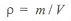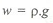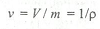# Important Terms Used in Hydraulics and Fluid Mechanics

Density or mass density, Weight density or specific weight, specific volume and specific gravity are important terms used in Hydraulics and Fluid Mechanics :

I. Density or mass density. It is defined as the mass per unit volume of a liquid at a standard temperature and pressure. It is usually denoted by p (rho). It is expressed in kg/m3. Mathematically, density or mass density,where

m = Mass of the liquid, and
V = Volume of the liquid.

2. Weight density or specific weight.
It is defined as the weight per unit volume of a liquid at a standard temperature and pressure. It is usually denoted by w. It is expressed in kN/m3 or N/m3 or N/mm3. Mathematically, weight density or specific weight,Note : For water, w = 9.81 kN / m3 = 9.81 x 103 N / m3 = 9.81 x 10-6 N / mm3.

3. Specific volume. It is defined as the volume per unit mass of the liquid. It is denoted by v. Mathematically, specific volume,4. Specific gravity. It is defined as the ratio of specific weight of a liquid to the specific weight of pure water at a standard temperature (4°C). It has no units.

Note: The specific gravity of pure water is taken as unity.

### You may like these posts:

1.2.